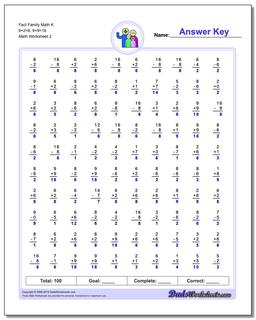# Math Worksheets: Fact Family Math: Fact Family Math: Fact Family Math K 6+2=8, 9+9=18 (Second Worksheet)## Fact Family Math K 6+2=8, 9+9=18 (Second Worksheet)

PropertyValue
DescriptionFact Family Math K 6+2=8, 9+9=18: These math worksheets have 100 addition and subtraction fact family problems and make for a challenging two minute test. (Second Worksheet)
Resource TypeWorksheet